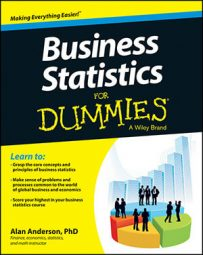The Poisson distribution is useful for measuring how many events may occur during a given time horizon, such as the number of customers that enter a store during the next hour, the number of hits on a website during the next minute, and so forth. The Poisson process takes place over time instead of a series of trials; each interval of time is assumed to be independent of all other intervals.

You calculate Poisson probabilities with the following formula: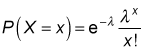Here's what each element of this formula represents: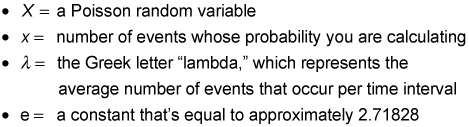e is a constant that's widely used in financial applications. One of the most important uses is in computing present values of sums of money when interest rates are continuously compounded — compounded an infinite number of times. Most calculators have a key labeled ex that you can use to calculate the value of e raised to a specified power. In Excel, the appropriate function for determining the value of e is EXP.

For example, suppose that the number of messages that a person receives on his cellphone averages one per hour and that the number of messages received each hour is independent of all other hours. What's the probability of his receiving two messages in the next hour?

In this case, the value of lambda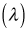is equal to 1, because the average number of messages each hour equals 1. The probability of receiving two messages during the next hour is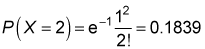Alternatively, you can get results from a Poisson table set up like this table.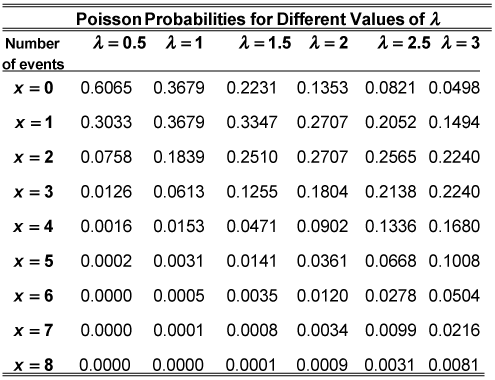The table shows the Poisson probabilities for different values of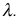In the cellphone example, because x = 2 and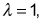the appropriate probability P(X = 2) is found in the 'x = 2' row and the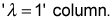The probability is 0.1839.

If you don't care for using formulas or a table, try a specialized calculator or Excel. For Excel 2007 and older versions, use the POISSON function; for Excel 2010, use the POISSON.DIST function.

The moments of the Poisson distribution are used to represent the average value of the distribution and the dispersion of the distribution. As with the binomial and geometric distribution, these moments may be computed with simplified formulas.